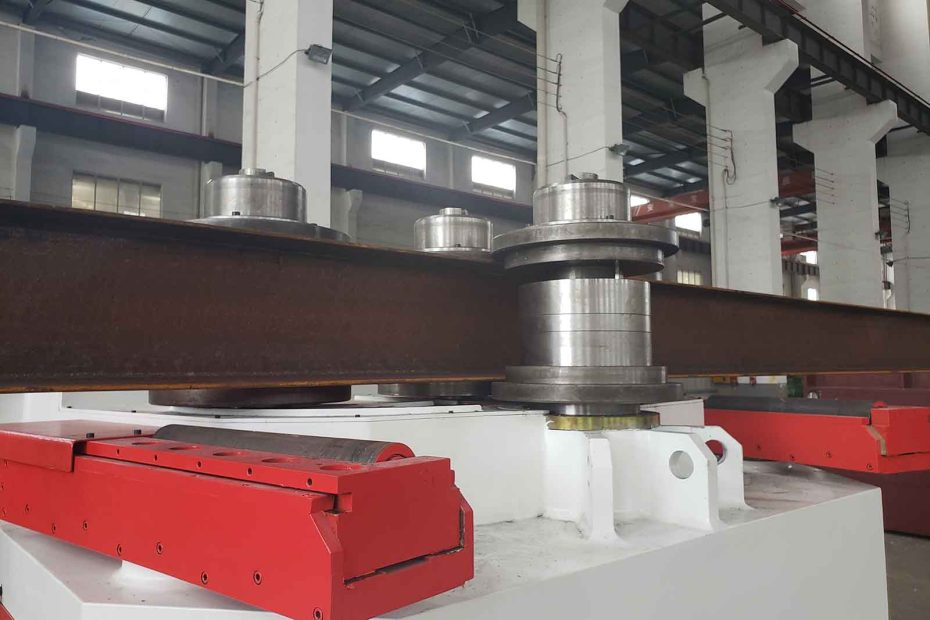# Understanding the Calculation of H-Beam BendingH-beams, also known as I-beams or universal beams, are structural steel members widely used in construction and engineering projects. Bending H-beams is a common requirement to achieve specific shapes or accommodate architectural and structural design needs. This article explores the calculation process involved in H-beam bending, providing insights into the key factors and considerations for successful bending operations.

## Calculating H-Beam Bending

Bending an H-beam involves determining the required bending moment, stress distribution, and the resulting deflection. These calculations are crucial for ensuring the structural integrity and performance of the beam during and after the bending process.

### 4 Factors Affecting H-Beam Bending

1. Material Properties: The material properties of the H-beam, such as its modulus of elasticity and yield strength, significantly influence the bending process. Different steel grades have varying resistance to deformation and require different bending approaches.
2. Bending Moment: The bending moment refers to the applied force that induces bending in the H-beam. It is crucial to calculate the bending moment accurately to determine the required bending capacity of the beam and select the appropriate bending equipment.
3. Section Modulus: The section modulus of an H-beam is a geometric property that represents its resistance to bending. It is calculated based on the dimensions and shape of the beam’s cross-section. The section modulus helps determine the maximum allowable bending stress and the beam’s ability to withstand the applied bending moment.
4. Load Distribution: Understanding the load distribution along the length of the H-beam is essential for calculating the bending moment accurately. Different loading conditions, such as uniformly distributed loads or concentrated loads, result in different bending moments and require different bending calculations.

## Calculation Methodology

The calculation of H-beam bending typically involves the following steps: